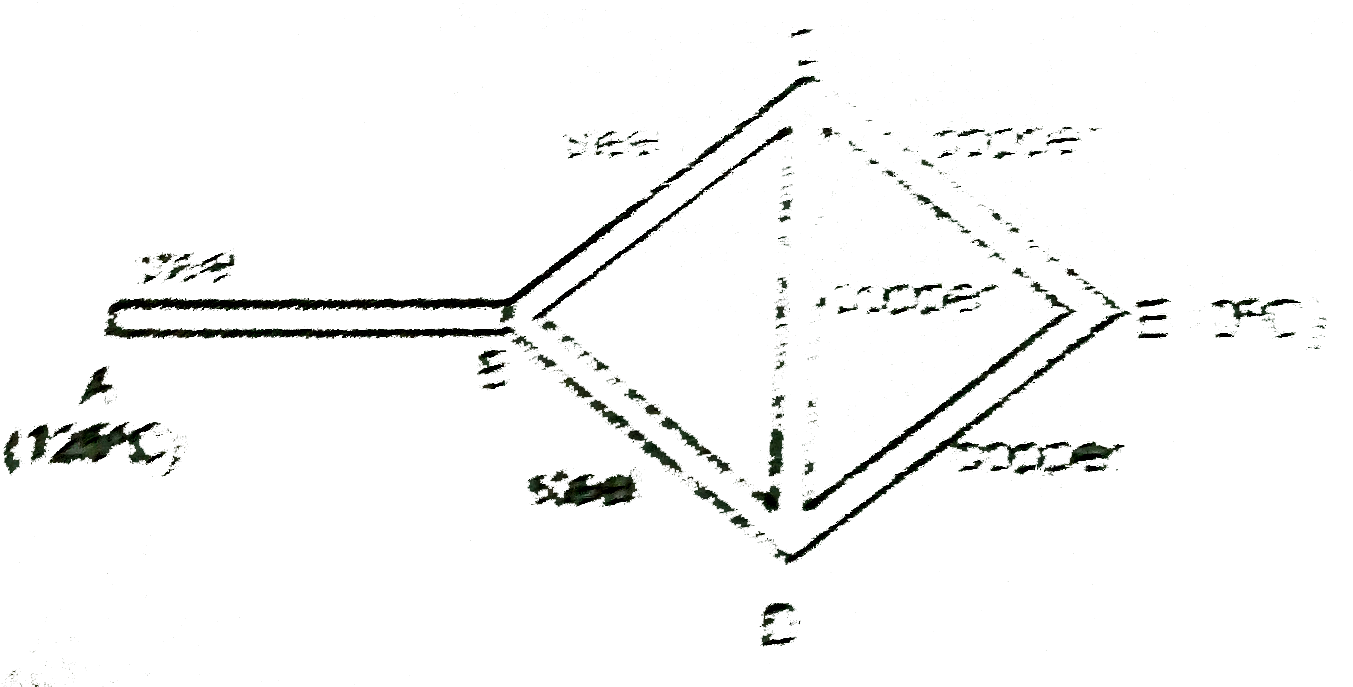# Three copper rods and three steel rods each of length l = 10 cm and area of cross-section 1cm^(2) are connected as shown If ends A and E are m

85 views
in Physics
closed
Three copper rods and three steel rods each of length l = 10 cm and area of cross-section 1cm^(2) are connected as shownIf ends A and E are maintained at temperatures 125^(@)C and 0^(@)C respectively, calculate the amount of heat flowing per second from the hot of cold function. [K_(Cu) = 400 W//m-K, K_(steel) = 50 W//m-K]

by (90.1k points)

R_("steel") = (L)/(KA) = (10^(-1)m)/(50(W//m-^(@)C)xx10^(-4)m^(2)) = (1000)/(50).^(@)C//W. Similarly R_(Cu) = (1000)/(400).^(@)C//W
Junction C and D are identical in every respect and both will have same temperature. Consequently. The rod CD is in thermal equilibrium and no heat will flow through it. Hence it can be neglected in further analysis.
Now rod BC and CE are in series their equilvalet resistance is R_(1) = R_(s) +R_(Cu) similarly rods BD and DE are in series with same equilvalent resistance R_(1) = R_(s) +R_(Cu) these two are in parallel giving an equilvalent resistance of
(R_(1))/(2) = (R_(S)+R_(Cu))/(2)
This resistance is connected in series with rod AB. Hence the net equilvalent of combination is
R = R_(steel) +(R_(1))/(2) (3R_(steel)+R_(Cu))/(2) = 500 ((3)/(50)+(1)/(400)).^(@)C//W
Now, i = (T_(H)-T_(C))/(R) =(125^(@)C)/(500((3)/(50)+(1)/(400)).^(@)C//W)=4watt.